# Dimensional Analysis Notes | Study Mechanical Engineering SSC JE (Technical) - Mechanical Engineering

## Mechanical Engineering: Dimensional Analysis Notes | Study Mechanical Engineering SSC JE (Technical) - Mechanical Engineering

The document Dimensional Analysis Notes | Study Mechanical Engineering SSC JE (Technical) - Mechanical Engineering is a part of the Mechanical Engineering Course Mechanical Engineering SSC JE (Technical).
All you need of Mechanical Engineering at this link: Mechanical Engineering

DIMENSIONAL ANALYSIS

• Velocity potential = [LT–1]
Stream function = [L2 T–1]
Acceleration = [LT–2]
Vorticity = [T–1] 
• Total no. of variables influencing the problem is equal to the no. of independent variables plus one, one being the no. of dependent variable. 
• Buckingham π theorem states that if all the n-variable are described by m fundamental dimensions, they may be grouped into (n - m) dimensions p terms. 
• Selection of 3 repeating variables from the geometry of flow, fluid properties and fluid motion. 
• Geometric similarity - similarity of shape
Kinematic similarity - similarity of motion
Dynamic similarity - similarity of forces
 Number                                 Equation                                       Significance Reynolds No.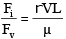Flow in closed conduit pipeFroude No.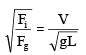where a free surface is present, structureEulers No.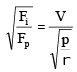In cavitation studies.Mach No.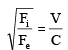where fluid compressibility is important.Weber No.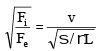In capillary studies.

 Reynolds Model Law :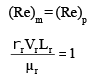(i) Velocity ratio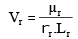(ii) Time ratio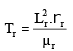(iii) Acceleration ratio,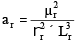(iv) Force ratio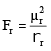(v) Power ratio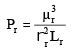(vi) Discharge ratio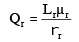Applications of Reynold’s Model Law :-

• Flow through small sized pipes 
• Low velocity motion around automobiles and aeroplane. 
• Submarines completely under water. 
• Flow through low speed trubo machines. 

Froude’s Model law :

(i) (Fr)prototype  =  (Fr)model
Vp / √ gL= Vm / √ gm Lp
It the place of model and prototype is same, then gm = gp
V =  √Lr
(ii) Time scale ratio
Tr  =  √ Lr
(iii) Acceleration scale ratio
ar  = 1
(iv) Discharge scale ratio
Qr  = Lr5/2
(v) Force scale ratio
F = ρp / ρm x (L/ Lm)x (Vp / Vm)2
If the fluid used in model and prototype is same, then
Fr  = Lr3
(vi) Pressure scale ratio
Pr  =  Lr

Applications :

• Open channels 
• Notches & weirs 
• Spill ways & dams 
• Liquid jets from orifice 
• Ship partially submerged in rough & turbulent sea
The document Dimensional Analysis Notes | Study Mechanical Engineering SSC JE (Technical) - Mechanical Engineering is a part of the Mechanical Engineering Course Mechanical Engineering SSC JE (Technical).
All you need of Mechanical Engineering at this link: Mechanical EngineeringUse Code STAYHOME200 and get INR 200 additional OFF

## Mechanical Engineering SSC JE (Technical)

6 videos|89 docs|55 tests

Track your progress, build streaks, highlight & save important lessons and more!

,

,

,

,

,

,

,

,

,

,

,

,

,

,

,

,

,

,

,

,

,

;